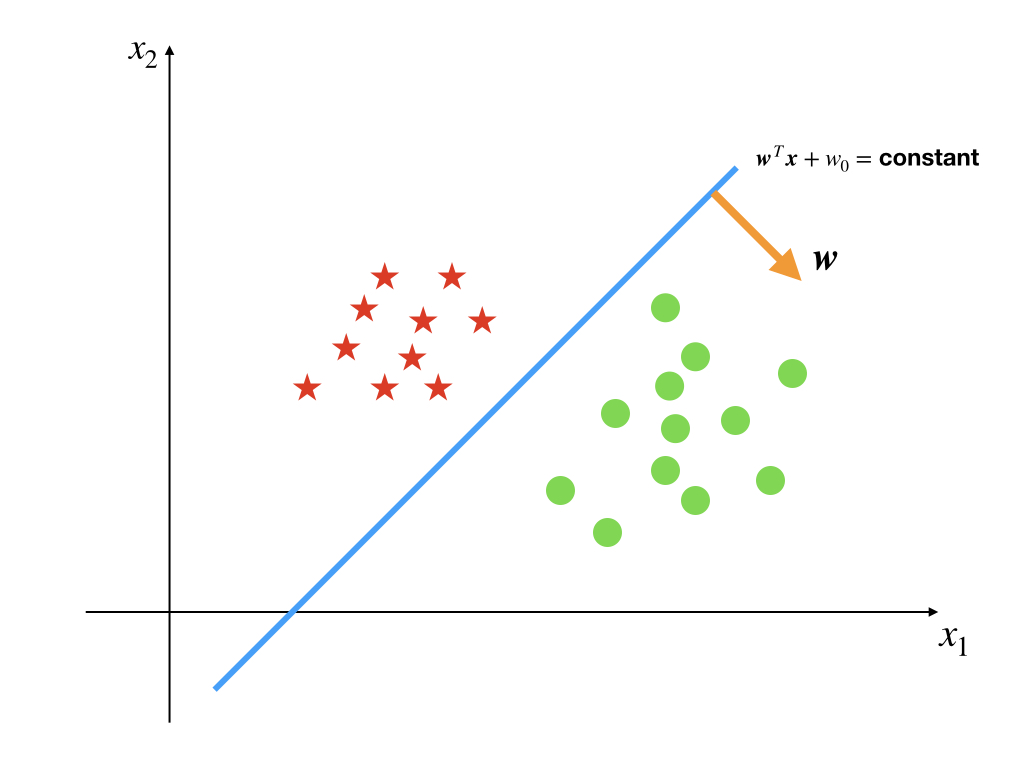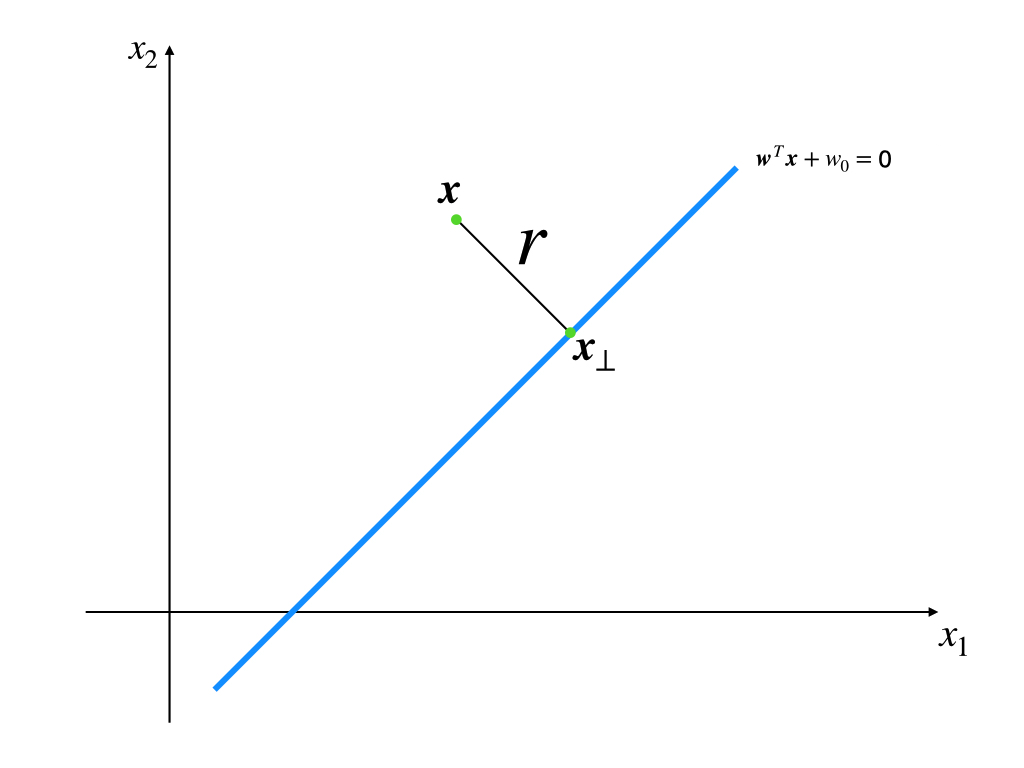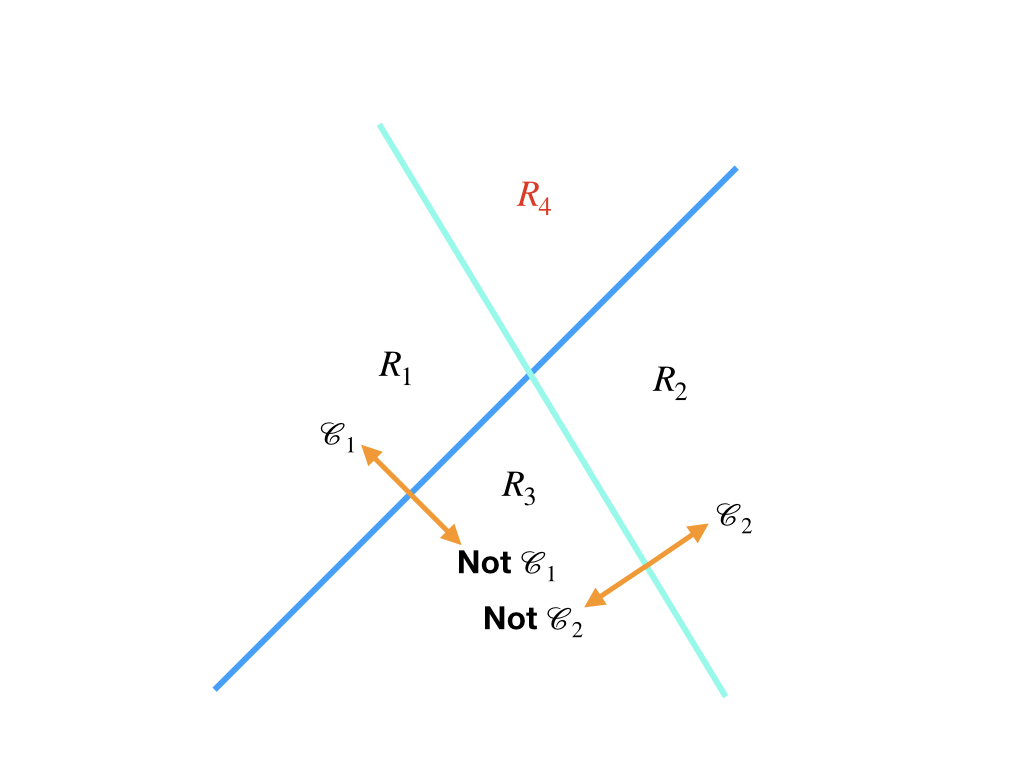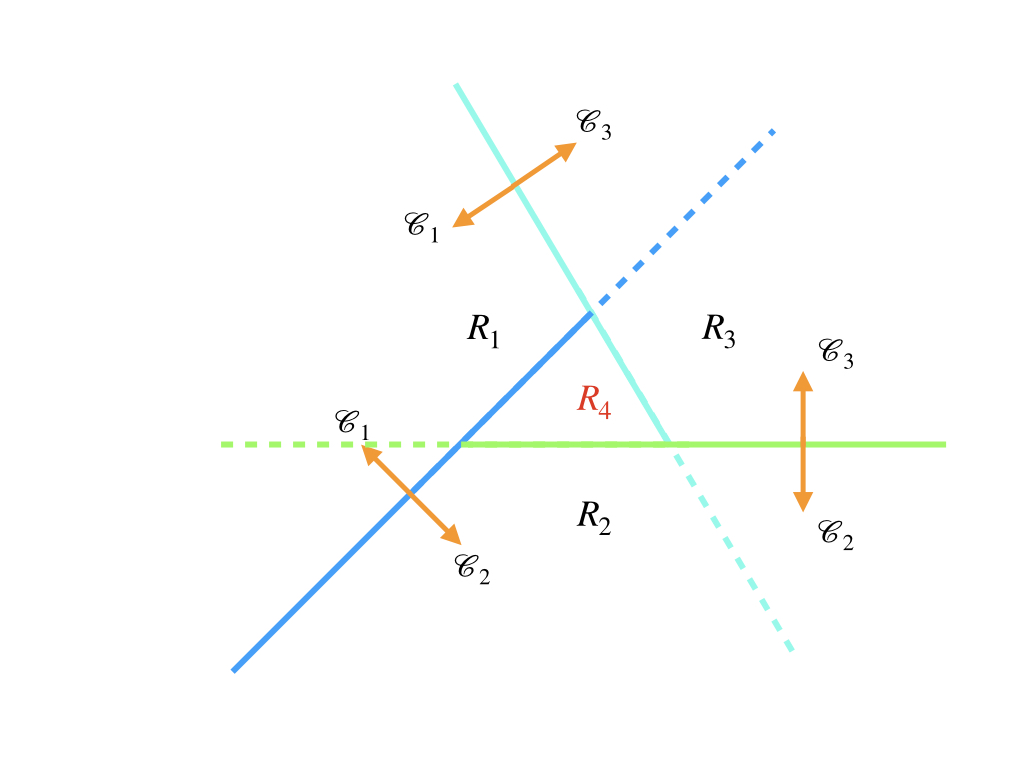## Preliminaries#

1. convex definition
2. linear algebra
• vector length
• vector direction

## Discriminant Function in Classification#

The discriminant function or discriminant model is on the other side of the generative model. And we, here, have a look at the behavior of the discriminant function in linear classification.1

In the post ‘Least Squares Classification’, we have seen, in a linear classification task, the decision boundary is a line or hyperplane by which we separate two classes. And if our model is based on the decision boundary or, in other words, we separate inputs by a function and a threshold, the model is a discriminant model and the decision boundary is formed by the function and a threshold.

Now, we are going to talk about what the decision boundaries look like in the $$K$$-classes problem when $$K=2$$ and $$K>2$$. To illustrate the boundaries, we only consider the 2D(two dimensional) input vector $$\mathbf{x}$$ who has only two components.

## Two classes#

The easiest decision boundary comes from 2-dimensional input space which is separated into 2 regions:whose decision boundary is:

$\mathbf{w}^T\mathbf{x}+w_0=\text{ constant }\tag{1}$

This equation is equal to $$\mathbf{w}^T\mathbf{x}+w_0=0$$ because $$w_0$$ is also a constant, so it can be merged with the r.h.s. constant. Of course, the 1-dimensional input space is easier than 2-dimensional, and its decision boundary is a point.

Let’s go back to the line, and it has the following properties:

1. The vector $$\mathbf{w}$$ always points to a certain region and is perpendicular to the line.
2. $$w_0$$ decides the location of the boundary relative to the origin.
3. The perpendicular distance $$r$$ to the line of a point $$\mathbf{x}$$ can be calculated by $$r=\frac{y(\mathbf{x})}{||\mathbf{w}||}$$ where $$y(\mathbf{x})=\mathbf{w}^T\mathbf{x}+w_0$$

Because these three properties are all basic concepts of a line, we just prove the third point roughly:

proof: We set $$\mathbf{x}_{\perp}$$ is the projection of $$\mathbf{x}$$ on the line.We using the first point that $$\mathbf{w}$$ is perpendicular to the line and $$\frac{\mathbf{w}}{||\mathbf{w}||}$$ is the union vector:

$\mathbf{x}=\mathbf{x}_{\perp}+r\frac{\mathbf{w}}{||\mathbf{w}||}\tag{2}$

and we substitute equation (2) to the line function $$y(\mathbf{x})=\mathbf{w}^T\mathbf{x}+w_0$$ :

\begin{aligned} y(\mathbf{x})&=\mathbf{w}^T(\mathbf{x}_{\perp}+r\frac{\mathbf{w}}{||\mathbf{w}||})+w_0\\ &=\mathbf{w}^T\mathbf{x}_{\perp}+\mathbf{w}^Tr\frac{\mathbf{w}}{||\mathbf{w}||}+w_0\\ &=\mathbf{w}^Tr\frac{\mathbf{w}}{||\mathbf{w}||}\\ &=r\frac{||\mathbf{w}||^2}{||\mathbf{w}||}\\ \end{aligned}\tag{3}

So we have

$r=\frac{y(\mathbf{x})}{||\mathbf{w}||}\tag{4}$

Q.E.D.

However, augmented vectors $$\mathbf{w}= \begin{bmatrix}w_0&w_1& \cdots&w_d\end{bmatrix}^T$$ and $$\mathbf{x}= \begin{bmatrix}1&x_1& \cdots&x_d\end{bmatrix}^T$$ can cancel $$w_0$$ of the original boundary equation. So a $$d+1$$-dimensional hyperplane that went through the origin could be instea replaced by an $$d$$-dimensional hyperplane.

## Multiple Classes#

Things changed when we consider more than 2 classes. Their boundaries become more complicated, and we have 3 different strategies for this problem intuitively:

### 1-versus-the-rest Classifier#

This strategy needs at least $$K-1$$ classifiers(boundaries). Each classifier $$k$$ just decides which side belongs to class $$k$$ and the other side does not belong to $$k$$. So when we have two boundaries, like:where the region $$R_4$$ is embarrassed, based on the properties of the decision boundary, and the definition of classification in the post‘From Linear Regression to Linear Classification’, region $$R_4$$ can not belong to $$\mathcal{C}_1$$ and $$\mathbb{C}_2$$ simultaneously.

So the first strategy can work for some regions, but there are some black whole regions where the input $$\mathbf{x}$$ belongs to more than one class and some white whole regions where the input $$\mathbf{x}$$ belongs to no classes(region $$R_3$$ could be such a region)

### 1-versus-1 classifier#

Another kind of multiple class boundary is the combination of several 1-versus-1 linear decision boundaries. Both sides of a decision boundary belong to a certain class, not like the 1-versus-rest classifier. And to a $$K$$ class task, it needs $$K(K-1)/2$$ binary discriminant functions.However, the contradiction still exists. Region $$R_4$$ belongs to class $$\mathcal{C}_1$$, $$\mathcal{C}_2$$, and $$\mathcal{C}_3$$ simultaneously.

So this is also not good for all situations.

### $$K$$ Linear functions#

We use a set of $$K$$ linear functions: \begin{aligned} y_1(\mathbf{x})&=\mathbf{w}^T_1\mathbf{x}+w_{10}\\ y_2(\mathbf{x})&=\mathbf{w}^T_2\mathbf{x}+w_{20}\\ &\vdots \\ y_K(\mathbf{x})&=\mathbf{w}^T_K\mathbf{x}+w_{K0}\\ \end{aligned}\tag{5}

and an input belongs to $$k$$ when $$y_k(\mathbf{x})>y_j(\mathbf{x})$$ where $$j\in \{1,2,\cdots,K\}$$ that $$j\neq k$$. According to this definition, the decision boundary between class $$k$$ and class $$j$$ is $$y_k(\mathbf{x})=y_j(\mathbf{x})$$ where $$k,j\in\{1,2,\cdots,K\}$$ and $$j\neq k$$. Then a decision hyperplane is defined as:

$(\mathbf{w}_k-\mathbf{w}_j)^T\mathbf{x}+(w_{k0}-w_{j0})=0\tag{6}$

These decision boundaries separate the input spaces into $$K$$ single connect, convex regions.

proof: choose two points in the region $$k$$ that $$k\in \{1,2,\cdots,K\}$$. $$\mathbf{x}_A$$ and $$\mathbf{x}_B$$ are two points in the region. An arbitrary point on the line between $$\mathbf{x}_A$$ and $$\mathbf{x}_B$$ can be written as $$\mathbf{x}'=\lambda \mathbf{x}_A + (1-\lambda)\mathbf{x}_B$$ where $$0\leq\lambda\leq1$$. For the linearity of $$y_k(\mathbf{x})$$ we have:

$y_k(\mathbf{x}')=\lambda y_k(\mathbf{x}_A) + (1-\lambda)y_k(\mathbf{x}_B)\tag{7}$

Because $$\mathbf{x}_A$$ and $$\mathbf{x}_B$$ belong to class $$k$$, $$y_k(\mathbf{x}_A)>y_j(\mathbf{x}_A)$$ and $$y_k(\mathbf{x}_B)>y_j(\mathbf{x}_B)$$ where $$j\neq k$$. Then $$y_k(\mathbf{x}')>y_j(\mathbf{x}')$$ and the region of class $$k$$ is convex.

Q.E.D

The last strategy seems good. And what we should do is estimate the parameters of the model. The most famous approaches that will study are: 1. Least square 2. Fisher’s linear discriminant 3. Perceptron algorithm

1. Bishop, Christopher M. Pattern recognition and machine learning. springer, 2006.↩︎